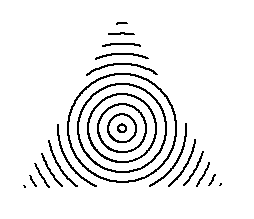# 三角圆图## 如本文章标有价格,需议价或其它事情商议请加微信pythonxia李兴球Python三角圆图

```"""
三角圆图.py
"""
import turtle

def draw_circle(pos,radius):
"""以pos为中心点为半径为radius的圆"""
pass

turtle.speed(0)
turtle.delay(0)
turtle.penup()
turtle.pensize(2)
# 画一个三角形，获取它们的顶点坐标
turtle.begin_poly()
turtle.fd(200)
turtle.lt(120)
turtle.fd(200)
turtle.lt(120)
turtle.fd(200)
turtle.lt(120)
turtle.end_poly()
p = list(turtle.get_poly())                # 转换成列表
p.pop()
# 求出三角形中心点坐标，以这个为圆心画圆
centerx = (p + p + p)/3
centery = (p + p + p)/3
center = centerx,centery

s = turtle.getscreen()
s.onclick(inside)
for radius in range(4,120,10):           # 画一些圆,超出三角范围则不画
draw_circle(center,radius)
turtle.ht()
turtle.done()

```

VIP免费查看

scratch